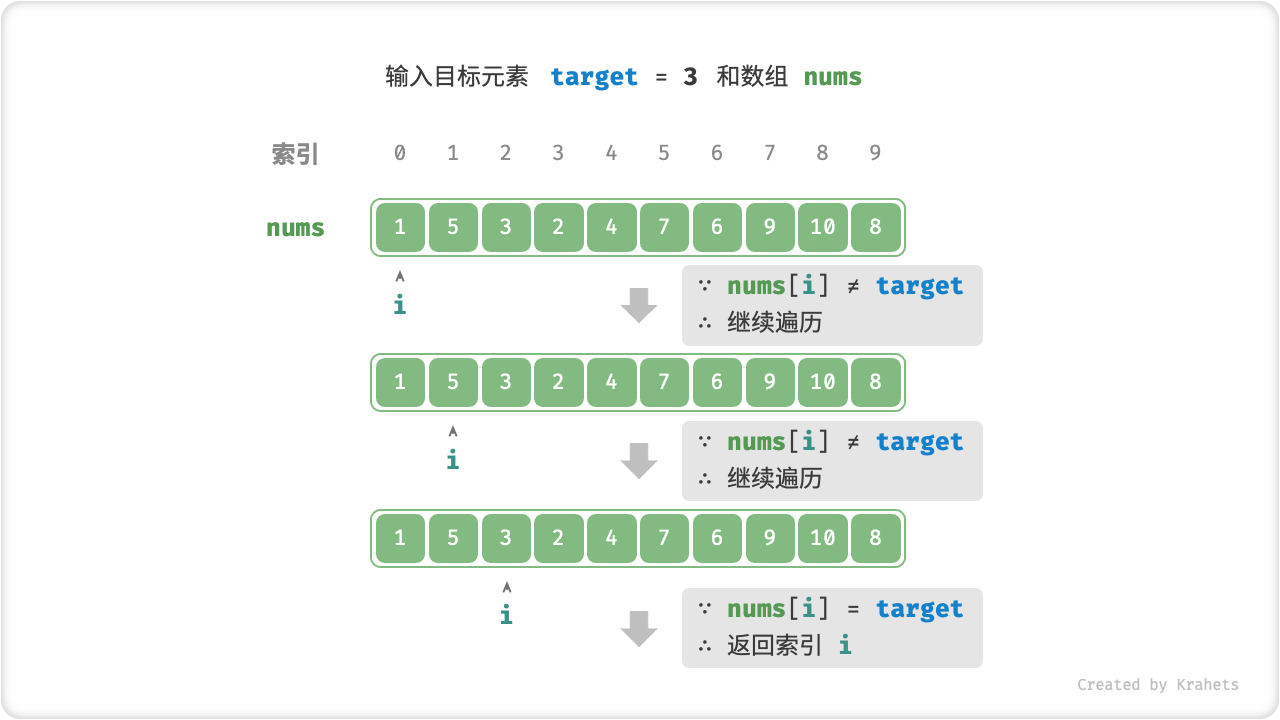# 线性查找¶

「线性查找 Linear Search」是一种最基础的查找方法，其从数据结构的一端开始，依次访问每个元素，直到另一端后停止。

## 算法实现¶linear_search.java
/* 线性查找（数组） */
int linearSearch(int[] nums, int target) {
// 遍历数组
for (int i = 0; i < nums.length; i++) {
// 找到目标元素，返回其索引
if (nums[i] == target)
return i;
}
// 未找到目标元素，返回 -1
return -1;
}

linear_search.cpp
/* 线性查找（数组） */
int linearSearch(vector<int>& nums, int target) {
// 遍历数组
for (int i = 0; i < nums.size(); i++) {
// 找到目标元素，返回其索引
if (nums[i] == target)
return i;
}
// 未找到目标元素，返回 -1
return -1;
}

linear_search.py
""" 线性查找（数组） """
def linear_search(nums, target):
# 遍历数组
for i in range(len(nums)):
if nums[i] == target:  # 找到目标元素，返回其索引
return i
return -1                  # 未找到目标元素，返回 -1

linear_search.go
/* 线性查找（数组） */
func linerSearchArray(nums []int, target int) int {
// 遍历数组
for i := 0; i < len(nums); i++ {
// 找到目标元素，返回其索引
if nums[i] == target {
return i
}
}
// 未找到目标元素，返回 -1
return -1
}

linear_search.js


linear_search.ts


linear_search.c


linear_search.cs



linear_search.java
/* 线性查找（链表） */
ListNode linearSearch(ListNode head, int target) {
// 遍历链表
// 找到目标结点，返回之
}
// 未找到目标结点，返回 null
return null;
}

linear_search.cpp
/* 线性查找（链表） */
ListNode* linearSearch(ListNode* head, int target) {
// 遍历链表
// 找到目标结点，返回之
}
// 未找到目标结点，返回 nullptr
return nullptr;
}

linear_search.py
""" 线性查找（链表） """
# 遍历链表
if head.val == target: # 找到目标结点，返回之
return None                # 未找到目标结点，返回 None

linear_search.go
/* 线性查找（链表）*/
func linerSearchLinkedList(node *ListNode, target int) *ListNode {
// 遍历链表
for node != nil {
// 找到目标结点，返回之
if node.Val == target {
return node
}
node = node.Next
}
// 未找到目标元素，返回 nil
return nil
}

linear_search.js


linear_search.ts


linear_search.c


linear_search.cs